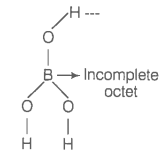Boric acid is an acid because its molecule

1. Contains replaceable H+ ion

2. Gives up a proton

3. Accepts OH- from water releasing proton

4. Combines with proton from water molecule

Hint: Boric acid, that is, H3BO3 is a lewis acid.

Lewis acids are substances whose octet is incomplete and accepts electrons.

Boric acid is a monobasic weak acid. It does not liberate H+ ion but accepts electrons from OH- ion i.e., behaves as Lewis acid.

${\mathrm{H}}_{3}{\mathrm{BO}}_{3}+{\mathrm{H}}_{2}\mathrm{O}\to \mathrm{B}{\left(\mathrm{OH}\right)}_{4}^{-}+{\mathrm{H}}^{+}$
or, $\mathrm{B}{\left(\mathrm{OH}\right)}_{3}+2{\mathrm{H}}_{2}\mathrm{O}\to {\left[\mathrm{B}{\left(\mathrm{OH}\right)}_{4}\right]}^{-}+{\mathrm{H}}_{3}{\mathrm{O}}^{+}$

The structure of H3BO3 is as shown below, where the octet of boron in H3BO3 is incomplete.